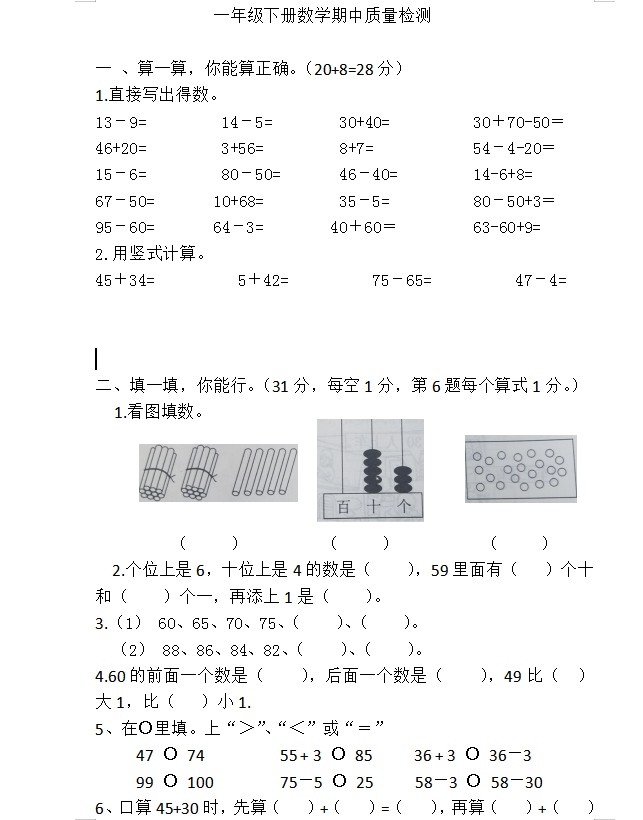1.直接写出得数。

13－9=       14－5=      30+40=          30＋70-50＝ 46+20=         3+56=         8+7=          54－4-20＝

15－6=       80－50=      46－40=         14-6+8=

67－50=      10+68=         35－5=          80－50+3＝

95－60=      64－3=        40＋60＝         63-60+9=